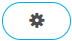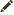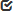# Use a Formula for Computing Job Execution Priority

Provide a formula that is used to calculate job execution priority.

You can choose to use a formula to sort jobs at the finest-granularity level. When the scheduler sorts jobs according to the formula, it computes a priority for each job. The priority computed for each job is the value produced by the formula. Jobs with a higher value get higher priority.

1. Click the Configure tab.
2. Choose the HPC to configure.
3. Click Scheduling from the PBS Professional menu located on the left-hand side of the web page.
4. Clicklocated in the upper right-hand corner of the web page.
5. Enable the Display Advanced Settings check box.
6. Clicklocated to the right of Job Sort Formula.
7. For Job Sort Formula, enter a formula to calculate job priority.
The formula can be made up of expressions, where expressions contain terms which are added, subtracted, multiplied, or divided, and which can contain parentheses, exponents, unary plus and minus, the ternary operator, and Python math module functions.
8. Clickto save the change.